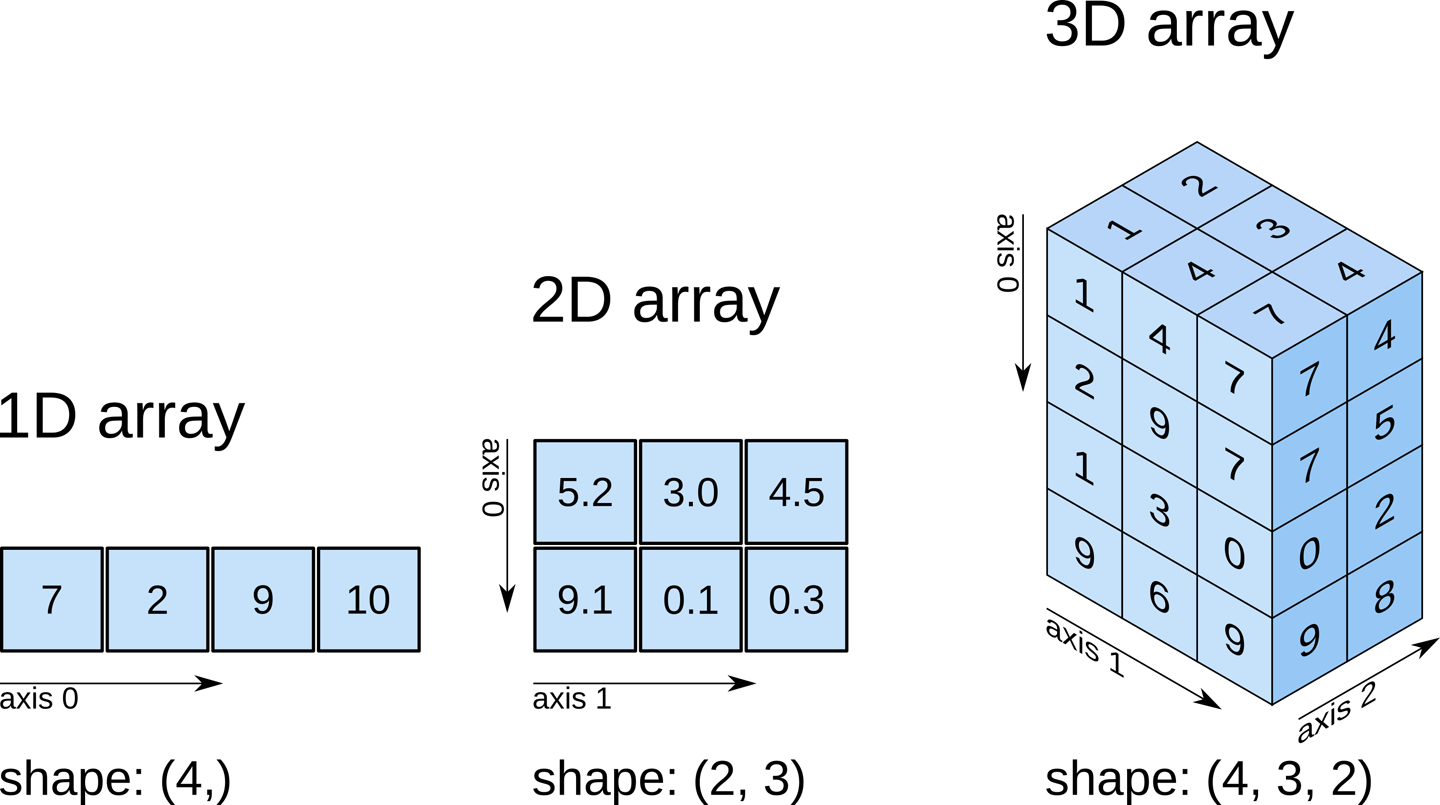Course Content

# Numpy Unveiled: Exploring the Powerhouse of Python

Numpy Unveiled: Exploring the Powerhouse of Python

##Importing NumPy and Creating NumPy Arrays

For this chapter, we will start from the basics. We will import the NumPy library and then we will create our very first `numPy` arrays. `NumPy` arrays are multi-dimensional arrays used for efficient numerical computing in Python. They are similar to Python's built-in list data type, but offer several benefits over lists:

`NumPy` arrays are more efficient for certain operations because they are designed to perform element-wise operations (e.g. addition, multiplication, etc.) more efficiently than lists.

`NumPy` arrays have a fixed size, which means that they cannot be resized like lists can. This can be beneficial because it allows NumPy to allocate memory more efficiently.

`NumPy` arrays have a fixed data type, which means that all elements in a `numPy` array must be of the same data type (e.g. all integers, all floating-point numbers, etc.). This can be beneficial because it allows `NumPy` to represent the data more efficiently in memory.`NumPy` arrays are commonly used in scientific computing and data analysis tasks because they allow you to perform operations on large amounts of data more efficiently than using lists or other data structures. `NumPy` provides many functions and methods for creating, manipulating, and performing operations on arrays, as well as for loading and saving array data to and from files.

1. Import the `numpy` library;
2. Create a 1D array (composed of numbers from 1 to 5);
3. Create a 2D array (2x3, numbers 1,2,3 on the first row and 4,5,6 on the second);
4. Create a `numpy` array with size 3x3 and fill it with zeros.

Everything was clear?

Section 1. Chapter 2## Start learning today and achieve coding mastery

• Master Python, SQL, JavaScript & more.
• Learn with Step-by-Step Lessons.
• Get Ready for Real-World Projects.
• Earn a Certificate Upon Completion.